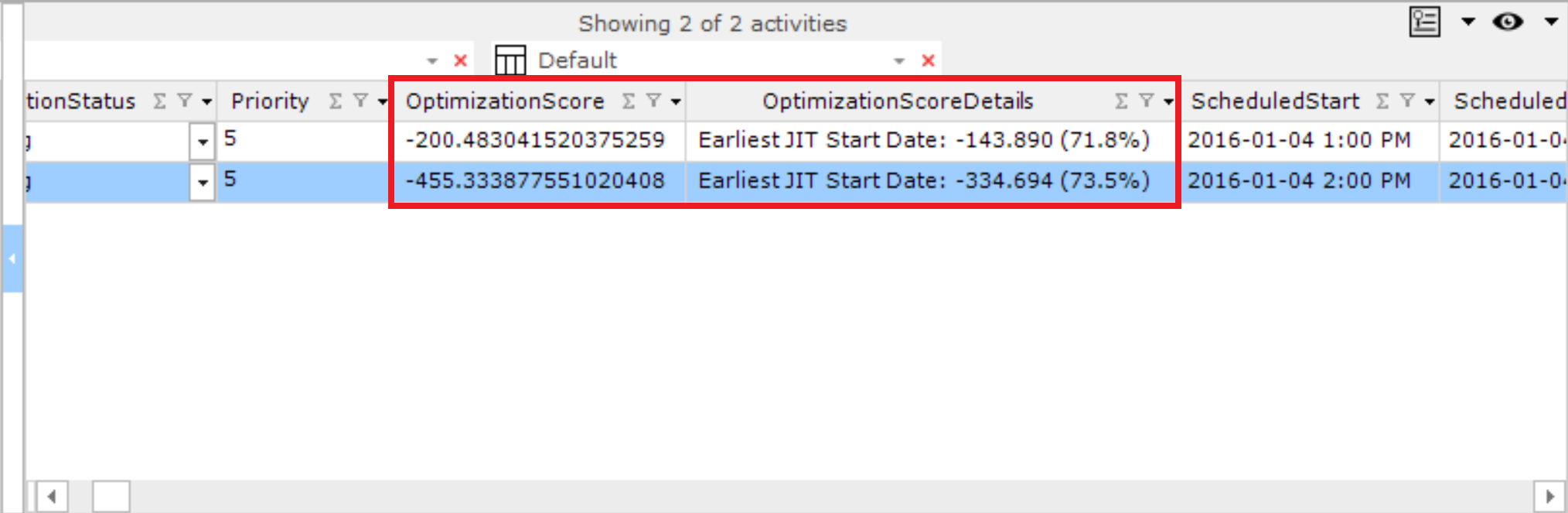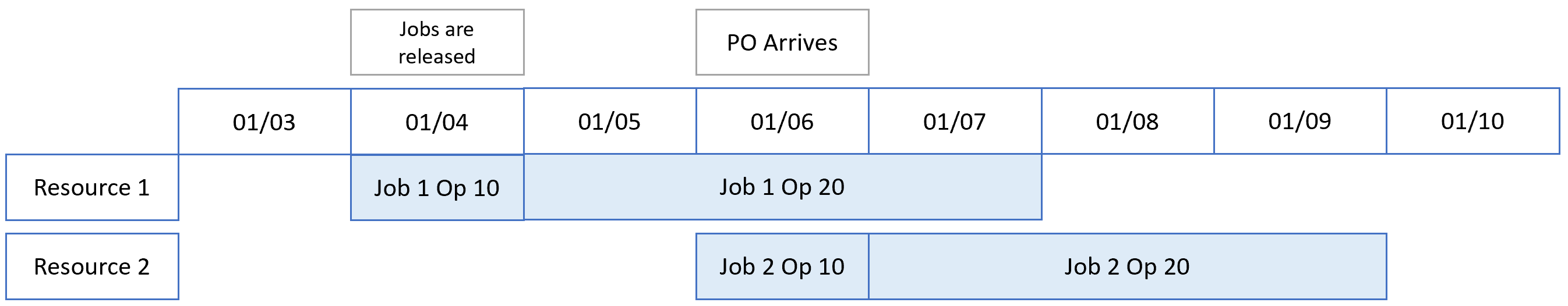# Optimize Score

#### Key Concepts

The Optimize Score is a numerical value that is calculated to give priority to certain activities when they are scheduled. Activities with higher Optimize Scores are selected to schedule earlier than those with lower scores (unless constraints are applied). The Score is the value at the simulated time during the optimization when the activity is scheduled.

The Optimize Score values can be seen in:

• The Activities View grid
• The Publish Database
• Labels
• The tool-tip window

The Optimize Score values are based on the last time the activity was evaluated for scheduling during an optimization. Additionally, the new fields are published and serialized so that they are preserved between sessions.

The Optimize Score and Score Details are displayed in the Activities View grid:• Optimization Score: This is the calculated value that will determine the priority of activities when scheduling.
• Optimization Score Details: This is a descriptive breakdown of the different factors such as Need Date, Priority, etc. that comprise the score. Each score details each factor and displays a percentage that indicates what fraction of the score the one factor makes up from the total points contributing to the score (positive and negative points). Only factors with a non-negative value are shown. Factors are sorted in order of decreasing percentages to show the highest-impact factors first.
• The Optimize Score Details are localized to the language of the PC. These values are stored in the scenario in the language they were created on the server. Starting the client will show the values as stored on the server and each optimization recreates the values in the user's language preference.

Note: Each factor value includes the impact of the factor's weight. For example, "Earliest Job NeedDate: 200 (66%), Lowest Priority Nbr: 100 (33%) would mean that the Earliest Job NeedDate contributed 200 points to the score and made up 66% of the total points (300).

#### Setting Up Optimize Scores:

Score calculation cab be enabled or disabled, To enable Optimize Score calculations, go to Settings | System Options | Scheduling then enable the "Calculate Optimize Scores" option.

Caution: Score calculation can consume hundreds of millions of score records and add significant time to an optimize. It is recommended to leave this option disabled to increase system performance.

Note: Many optimize scores change over time, making it possible for activities with lower scores to be scheduled earlier than activities with higher scores. For example, when using date-related factors, the scores may change from when the optimization was simulated as constraints or other factors may have changed.

#### How Optimize Scores are Calculated

The following example illustrates how PlanetTogether would create a schedule that it optimized based on different factors:

Starting Data:

• Two Resources: Resource 1 and Resource 2, each with the same Capability.
• Two Jobs:
• Job 1
• Need By 01/15
• Priority = 1
• Two Operations:
• Op 10: 1 day of work, requires 1 unit of Material A.
• Op 20: 3 days of work.
• Job 2
• Need By 01/15
• Priority = 3
• Two Operations:
• Op 10: 1 day of work, requires 1 unit of Material A.
• Op 20: 3 days of work.
• Inventory
• 1 unit of Material A is On-Hand.
• 1 unit of Material A is On-Order with a purchase order due on 01/06.
• Optimize Parameters:
• Release Rule: JIT with 7 days slack
• Current Clock Date: 01/01
• Optimize Rule: 50% weight on Need Date and 50% weight on Priority.

Step-By-Step Optimize Process:

1. Release Dates are calculated for each job. Since both jobs have the same need date (01/15) and the same work hours (4 days total), their JIT release dates are identical = 01/04. (Need Date of 01/15 minus 4 days of work minus 7 days of slack).
2. The first "event" to occur is the release of the two jobs on 01/04. The result of this event is that Op 10 for both jobs is added to the work queues for both resources.
3. The next event is for the resource with the highest Id to review its queue and see if any work can be scheduled at the simulated event time.
4. This resource calculates a "score" for the operations in its queue. The results are:
1. Job 1, Op 10 Score = 11 (Need Date minus event date) x 50% + 1 (Priority) x 50% = 6
2. Job 2, Op 10 Score = 11 (Need Date minus event date) x 50% + 3 (Priority) x 50% = 7
5. Job 1 has a lower score and is selected first by Resource 1 since it was the first resource to make a selection. Since there are enough materials on-hand, the operation is scheduled to run on 01/04. The material is allocated to Job 1, reducing the on-hand material to zero. No other operations can schedule at this time, and since this is the only operation that can start (Job 2 is still awaiting material), it is scheduled to start on 01/04.
6. The next event is the completion of Job 1 Op 10 on 01/05. This causes Resource 1 to become available and it causes Job 1 Op 20 to become ready and added to the queues. The scores are once again computed, and since this is the only operation that can start (Job 2 Op 10 is still waiting on materials), it is scheduled to start on 01/05.
7. The next event is the arrival of the purchase order on 01/06. This triggers the resources to evaluate their queues. At this point, Job 2 Op 10 can start since the material was received. Since Resource 2  is the only idle resource at this time, it selects Job 2 Op 10 and schedules it to run on 01/06.
8. The next event is the completion of Job 2 Op 10 which causes Op 20 to become ready and to schedule, completing the scheduling process.

The events and resulting schedule are illustrated below:Note: Each time optimize is performed, the JIT Start Dates are recalculated based on the resource the activities are scheduled on, and the actual setup times determined. Since JIT dates change, subsequent use of the optimize can potentially generate different schedules. To change this, go to: Settings | System Options | Scheduling and disable the option "Recalculate JIT on Schedule Change". This will make the JIT dates remain static after the initial optimize and will prevent optimization from recalculating the JIT start dates again and again.